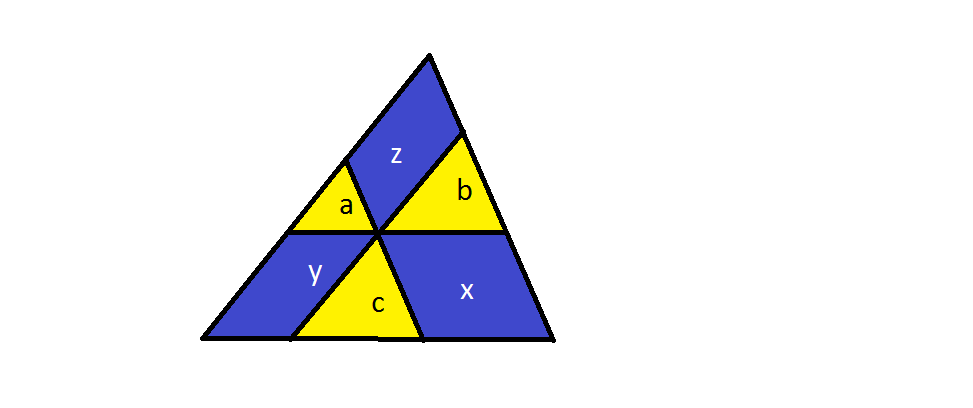# Conceptual Geometry

Geometry Level 4Through a point inside a triangle three lines parallel to the triangle’s sides are drawn.

Denote the areas of the parts into which these lines divide the triangle as plotted in the figure.

Find the least value of $\frac{a}{x} + \frac{b}{y} + \frac{c}{z}$.

×

Problem Loading...

Note Loading...

Set Loading...